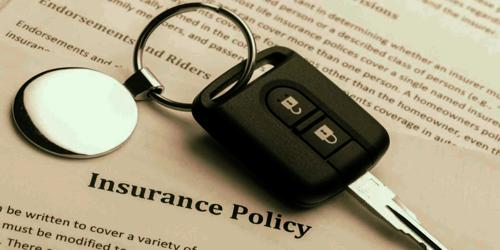# Various Classes of Rating

Various Classes of Rating

Class rating is a type of manual rating that places similar consumers into the same underwriting category. The second type of rating method is class rating. The class rating means that exposures with similar characteristics are placed in the same underwriting class and each is charged the same rate.

The pure premium can be determined by dividing the dollar amount incurred losses and loss-adjustment expense by the number of exposure units. Incurred losses include all losses paid during the accounting period, plus amounts held as reserves for the future payment of losses that have already occurred during the same period. Thus incurred losses include all losses that occur during the accounting period whether or not they have been paid by the end of the period. Loss adjustment expenses are the expense incurred by the company in adjusting losses during the same accounting period. The following example will illustrate how the pure premium is derived.

Example:

Assume that in auto collision insurance 500,000 automobiles is a given underwriting class generates incurred losses and loss-adjustment expense of \$ 30 million over a one year period. The pure premium is \$ 60. This can be illustrated by the following equation.

Pure premium = [Incurred losses and loss adjustment expense / Number of exposure units]

= [\$ 30,000,000 / 5,00,000] = \$ 60

The final step is to add a loading for an expense, underwriting profit, and a margin for contingencies. The expense loading is usually expressed as a percentage of the gross rate and is called the expense ratio. The final gross rate can be determined by dividing the pure premium by 1 minus the expense ratio. For example, if an expense is 40% of the gross rate, the final gross rate is \$ 100. This can be illustrated by the following equation.

Gross rate = [Pure premium / (1 – Expense ratio)]

= \$ 60 / (1 – 0.40) = \$ 100.

(B) Loss ratio method:

Under the loss tunic method, the actual loss ratio is compared with the expected loss ratio, and the rate is adjusted accordingly. The actual loss ratio is the ratio of incurred losses and loss-adjustment expense to earned premiums. The expected loss ratio is the percentage of the premiums that are expected to be used to pay losses. For example, assume that a line of insurance has incurred losses and loss-adjustment expense in the amount of \$ 800,000 and earned premiums are \$ 1 million. The actual loss ratio is 0.80, or 80 percent. If the expected losses are 0.70 or 70 per cent the rate must be increased by 14.3 percent. This can be illustrated by the following:

A-E 0.80-0.70

Rate change = (A – E) / R = (0.80 – 0.70) / 0.70 = 0.143 or 14.3%